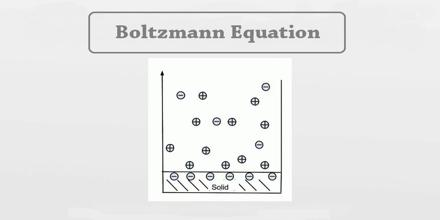Mathematic

# Boltzmann EquationBoltzmann Equation use in modern methods for solving partial differential equations and harmonic analysis to develop an analytical solution method and prove the global existence of classical solutions and rapid time decay to equilibrium for the long-range interactions. Boltzmann Equation is not a single equation but a family of equations, where one obtains different equations depending on the nature of the interaction between particles. It can be used to determine how physical quantities change, such as heat energy and momentum, when a fluid is in transport, and other properties characteristic to fluids such as viscosity, thermal conductivity, and electrical conductivity can be derived.